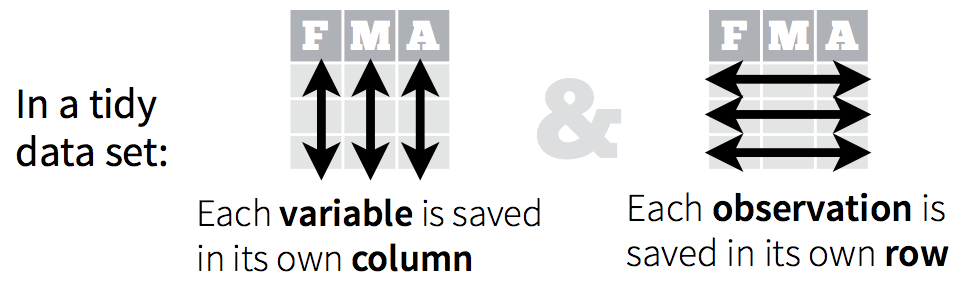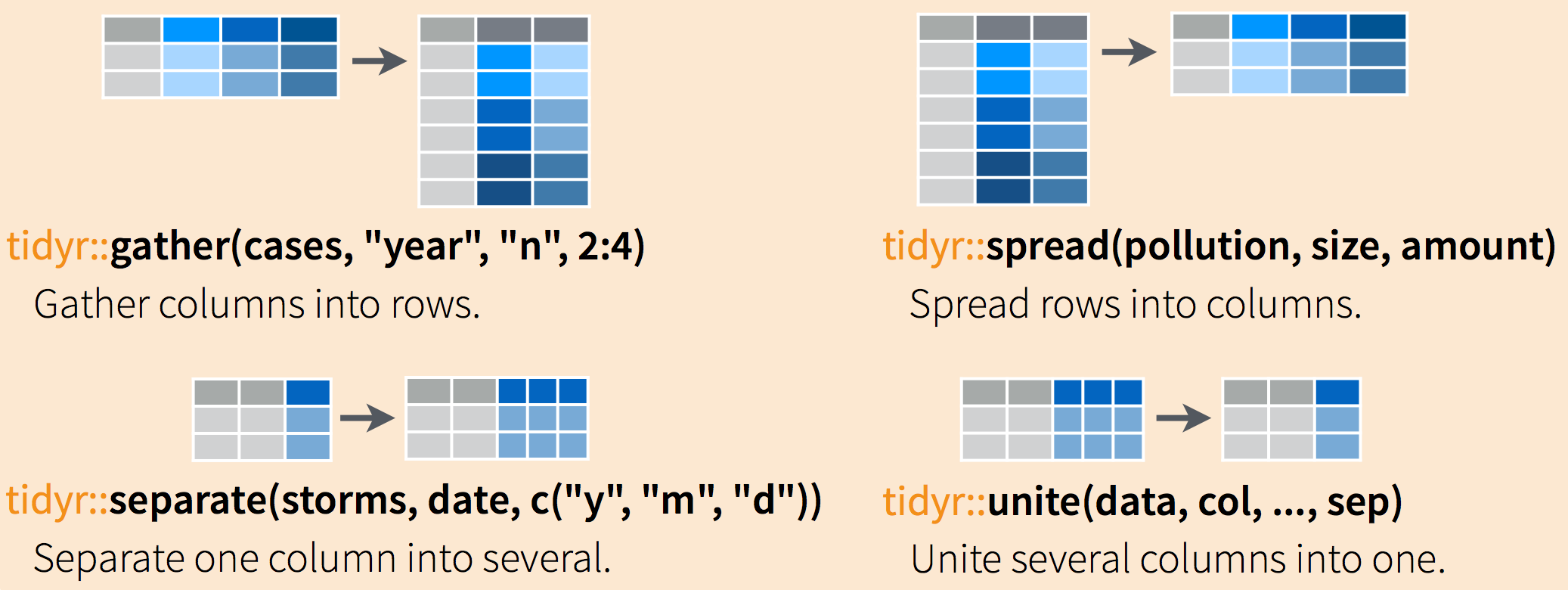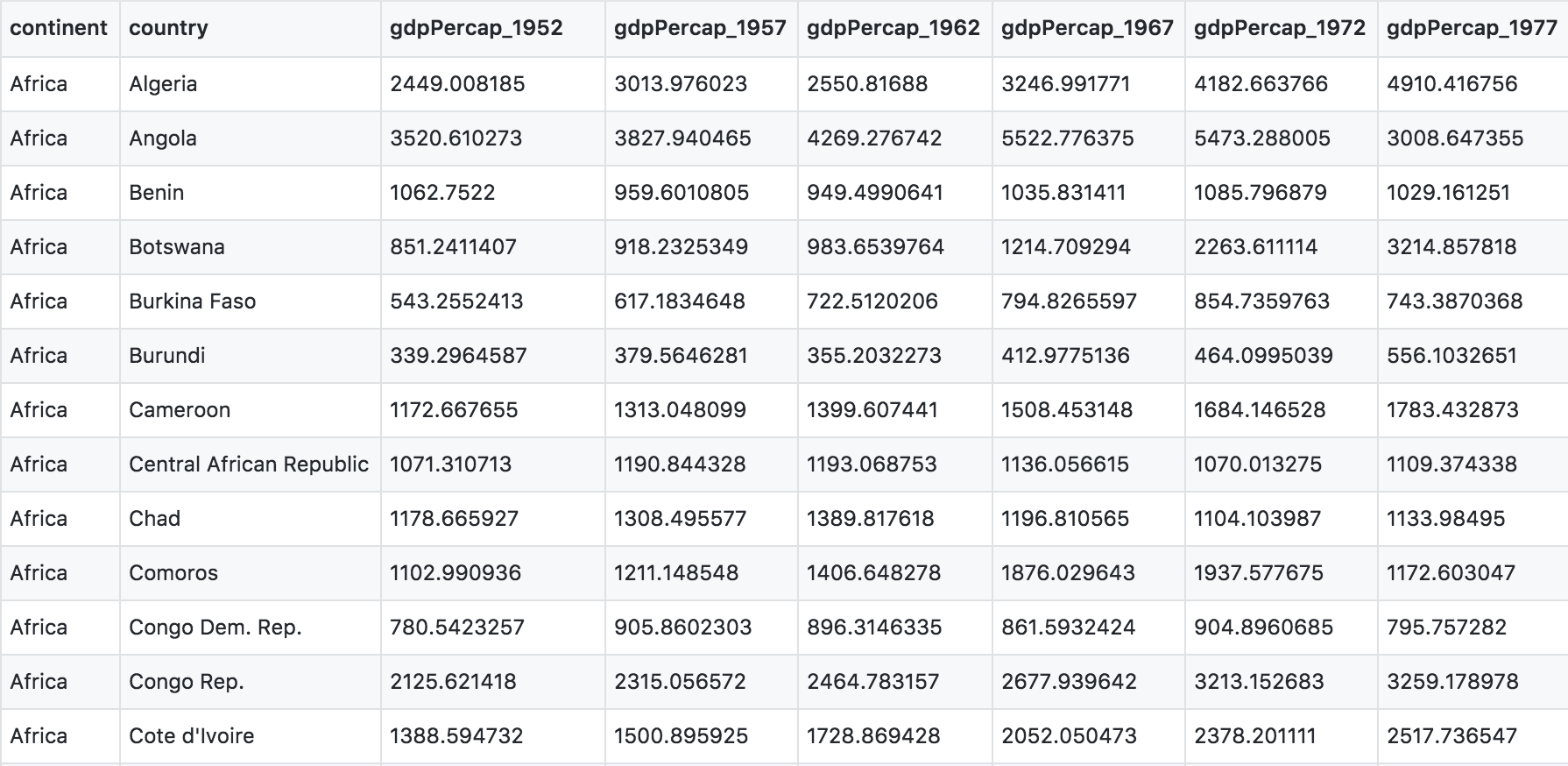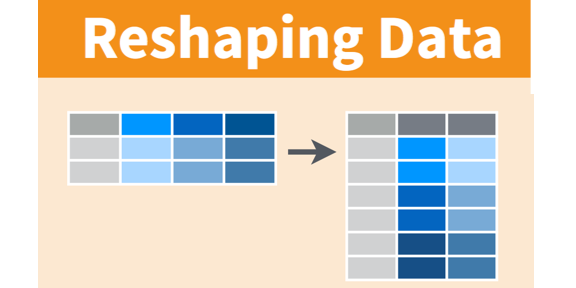# Chapter 7 Data Wrangling: `tidyr`

## 7.1 Objectives & Resources

Now you have some experience working with tidy data and seeing the logic of wrangling when data are structured in a tidy way. But ‘real’ data often don’t start off in a tidy way, and require some reshaping to become tidy. The `tidyr` package is for reshaping data. You won’t use `tidyr` functions as much as you use `dplyr` functions, but it is incredibly powerful when you need it.

### 7.1.1 Objectives

• learn `tidyr` with the `gapminder` package
• practice the RStudio-GitHub workflow
• your turn: use the data wrangling cheat sheet to explore window functions

### 7.1.2 Resources

These materials borrow heavily from:

### 7.1.3 Data and packages

We’ll use the package `tidyr` and `dplyr`, which are bundled within the `tidyverse` package.

We’ll also be using the Gapminder data we used when learning `dplyr`. We will also explore several datasets that come in Base R, in the `datasets` package.

## 7.2`tidyr` basics

Remember, from the `dplyr` section, that tidy data means all rows are an observation and all columns are variables.Why is this important? Well, if your data are formatted in a standard way, you will be able to use analysis tools that operate on that standard way. Your analyses will be streamlined and you won’t have to reinvent the wheel every time you see data in a different.

Let’s take a look at some examples.

Data are often entered in a wide format where each row is often a site/subject/patient and you have multiple observation variables containing the same type of data.

An example of data in a wide format is the `AirPassengers` dataset which provides information on monthly airline passenger numbers from 1949-1960. You’ll notice that each row is a single year and the columns are each month Jan - Dec.

``AirPassengers``
``````##      Jan Feb Mar Apr May Jun Jul Aug Sep Oct Nov Dec
## 1949 112 118 132 129 121 135 148 148 136 119 104 118
## 1950 115 126 141 135 125 149 170 170 158 133 114 140
## 1951 145 150 178 163 172 178 199 199 184 162 146 166
## 1952 171 180 193 181 183 218 230 242 209 191 172 194
## 1953 196 196 236 235 229 243 264 272 237 211 180 201
## 1954 204 188 235 227 234 264 302 293 259 229 203 229
## 1955 242 233 267 269 270 315 364 347 312 274 237 278
## 1956 284 277 317 313 318 374 413 405 355 306 271 306
## 1957 315 301 356 348 355 422 465 467 404 347 305 336
## 1958 340 318 362 348 363 435 491 505 404 359 310 337
## 1959 360 342 406 396 420 472 548 559 463 407 362 405
## 1960 417 391 419 461 472 535 622 606 508 461 390 432``````

This format is intuitive for data entry, but less so for data analysis. If you wanted to calculate the monthly mean, where would you put it? As another row?

Often, data must be reshaped for it to become tidy data. What does that mean? There are four main verbs we’ll use, which are essentially pairs of opposites:

• turn columns into rows (`gather()`),
• turn rows into columns (`spread()`),
• turn a character column into multiple columns (`separate()`),
• turn multiple character columns into a single column (`unite()`)## 7.3 Explore gapminder dataset.

Yesterday we started off with the gapminder data in a format that was already tidy. But what if it weren’t? Let’s look at a different version of those data.

The data are on GitHub. Navigate there by going to:

github.com > ohi-science > data-science-training > data > gapminder_wide.csv

or by copy-pasting this in the browser: `https://github.com/OHI-Science/data-science-training/blob/master/data/gapminder_wide.csv`

First have a look at the data.

You can see there are a lot more columns than the version we looked at before. This format is pretty common, because it can be a lot more intuitive to enter data in this way.Sometimes, as with the gapminder dataset, we have multiple types of observed data. It is somewhere in between the purely ‘long’ and ‘wide’ data formats:

• 3 “ID variables” (`continent`, `country`, `year`)
• 3 “Observation variables” (`pop`,`lifeExp`,`gdpPercap`).

It’s pretty common to have data in this format in most cases despite not having ALL observations in 1 column, since all 3 observation variables have different units. But we can play with switching it to long format and wide to show what that means (i.e. long would be 4 ID variables and 1 observation variable).

But we want it to be in a tidy way so that we can work with it more easily. So here we go.

You use `spread()` and `gather()` to transform or reshape data between wide to long formats.

### 7.3.1 Setup

OK let’s get going.

We’ll learn `tidyr` in an RMarkdown file within a GitHub repository so we can practice what we’ve learned so far. You can either continue from the same RMarkdown as yesterday, or begin a new one.

Here’s what to do:

1. Clear your workspace (Session > Restart R)
2. New File > R Markdown…, save as something other than `gapminder-wrangle.Rmd` and delete irrelevant info, or just continue using `gapminder-wrangle.Rmd`

I’m going to write this in my R Markdown file:

``Data wrangling with `tidyr`, which is part of the tidyverse. We are going to tidy some data!``

### 7.3.2 load `tidyverse` (which has `tidyr` inside)

First load `tidyr` in an R chunk. You already have installed the tidyverse, so you should be able to just load it like this (using the comment so you can run `install.packages("tidyverse")` easily if need be):

``library(tidyverse) # install.packages("tidyverse")``

## 7.4`gather()` data from wide to long formatRead in the data from GitHub. Remember, you need to click on the ‘Raw’ button first so you can read it directly. Let’s also read in the gapminder data from yesterday so that we can use it to compare later on.

``````## wide format
gap_wide <- readr::read_csv('https://raw.githubusercontent.com/OHI-Science/data-science-training/master/data/gapminder_wide.csv')

## yesterday's format
gapminder <- readr::read_csv('https://raw.githubusercontent.com/OHI-Science/data-science-training/master/data/gapminder.csv')``````

Let’s have a look:

``````head(gap_wide)
str(gap_wide)``````

While wide format is nice for data entry, it’s not nice for calculations. Some of the columns are a mix of variable (e.g. “gdpPercap”) and data (“1952”). What if you were asked for the mean population after 1990 in Algeria? Possible, but ugly. But we know it doesn’t need to be so ugly. Let’s tidy it back to the format we’ve been using.

Question: let’s talk this through together. If we’re trying to turn the `gap_wide` format into `gapminder` format, what structure does it have that we like? And what do we want to change?

• We like the continent and country columns. We won’t want to change those.
• We want 1 column identifying the variable name (`tidyr` calls this a ‘key’), and 1 column for the data (`tidyr` calls this the ’value’).
• We actually want 3 different columns for variable: `gdpPercap`, `lifeExp`, and `pop`.
• We would like year as a separate column.

Let’s get it to long format. We’ll have to do this in 2 steps. The first step is to take all of those column names (e.g. `lifeExp_1970`) and make them a variable in a new column, and transfer the values into another column. Let’s learn by doing:

Let’s have a look at `gather()`’s help:

``?gather``

Question: What is our key-value pair?

We need to name two new variables in the key-value pair, one for the key, one for the value. It can be hard to wrap your mind around this, so let’s give it a try. Let’s name them `obstype_year` and `obs_values`.

Here’s the start of what we’ll do:

``````gap_long <- gap_wide %>%
gather(key   = obstype_year,
value = obs_values)``````

Although we were already planning to inspect our work, let’s definitely do it now:

``str(gap_long)``
``````## Classes 'tbl_df', 'tbl' and 'data.frame':    5396 obs. of  2 variables:
##  \$ obstype_year: chr  "continent" "continent" "continent" "continent" ...
##  \$ obs_values  : chr  "Africa" "Africa" "Africa" "Africa" ...``````
``head(gap_long)``
``````## # A tibble: 6 x 2
##   obstype_year obs_values
##   <chr>        <chr>
## 1 continent    Africa
## 2 continent    Africa
## 3 continent    Africa
## 4 continent    Africa
## 5 continent    Africa
## 6 continent    Africa``````
``tail(gap_long)``
``````## # A tibble: 6 x 2
##   obstype_year obs_values
##   <chr>        <chr>
## 1 pop_2007     9031088
## 2 pop_2007     7554661
## 3 pop_2007     71158647
## 4 pop_2007     60776238
## 5 pop_2007     20434176
## 6 pop_2007     4115771``````

We have reshaped our dataframe but this new format isn’t really what we wanted.

What went wrong? Notice that it didn’t know that we wanted to keep `continent` and `country` untouched; we need to give it more information about which columns we want reshaped. We can do this in several ways.

One way is to identify the columns is by name. Listing them explicitly can be a good approach if there are just a few. But in our case we have 30 columns. I’m not going to list them out here since there is way too much potential for error if I tried to list `gdpPercap_1952`, `gdpPercap_1957`, `gdpPercap_1962` and so on. But we could use some of `dplyr`’s awesome helper functions — because we expect that there is a better way to do this!

``````gap_long <- gap_wide %>%
gather(key   = obstype_year,
value = obs_values,
dplyr::starts_with('pop'),
dplyr::starts_with('lifeExp'),
dplyr::starts_with('gdpPercap'))  #here i'm listing all the columns to use in gather

str(gap_long)
head(gap_long)
tail(gap_long)``````

Success! And there is another way that is nice to use if your columns don’t follow such a structured pattern: you can exclude the columns you don’t want.

``````gap_long <- gap_wide %>%
gather(key   = obstype_year,
value = obs_values,
-continent, -country)

str(gap_long)
head(gap_long)
tail(gap_long)``````

To recap:

Inside `gather()` we first name the new column for the new ID variable (`obstype_year`), the name for the new amalgamated observation variable (`obs_value`), then the names of the old observation variable. We could have typed out all the observation variables, but as in the `select()` function (see `dplyr` lesson), we can use the `starts_with()` argument to select all variables that starts with the desired character string. Gather also allows the alternative syntax of using the `-` symbol to identify which variables are not to be gathered (i.e. ID variables).

OK, but we’re not done yet. `obstype_year` actually contains two pieces of information, the observation type (`pop`,`lifeExp`, or `gdpPercap`) and the `year`. We can use the `separate()` function to split the character strings into multiple variables.

`?separate` –> the main arguments are `separate(data, col, into, sep ...)`. So we need to specify which column we want separated, name the new columns that we want to create, and specify what we want it to separate by. Since the `obstype_year` variable has observation types and years separated by a `_`, we’ll use that.

``````gap_long <- gap_wide %>%
gather(key   = obstype_year,
value = obs_values,
-continent, -country) %>%
separate(obstype_year,
into = c('obs_type','year'),
sep = "_",
convert = TRUE) #this ensures that the year column is an integer rather than a character``````

No warning messages…still we inspect:

``str(gap_long)``
``````## Classes 'tbl_df', 'tbl' and 'data.frame':    5112 obs. of  5 variables:
##  \$ continent : chr  "Africa" "Africa" "Africa" "Africa" ...
##  \$ country   : chr  "Algeria" "Angola" "Benin" "Botswana" ...
##  \$ obs_type  : chr  "gdpPercap" "gdpPercap" "gdpPercap" "gdpPercap" ...
##  \$ year      : int  1952 1952 1952 1952 1952 1952 1952 1952 1952 1952 ...
##  \$ obs_values: num  2449 3521 1063 851 543 ...``````
``head(gap_long)``
``````## # A tibble: 6 x 5
##   continent country      obs_type   year obs_values
##   <chr>     <chr>        <chr>     <int>      <dbl>
## 1 Africa    Algeria      gdpPercap  1952      2449.
## 2 Africa    Angola       gdpPercap  1952      3521.
## 3 Africa    Benin        gdpPercap  1952      1063.
## 4 Africa    Botswana     gdpPercap  1952       851.
## 5 Africa    Burkina Faso gdpPercap  1952       543.
## 6 Africa    Burundi      gdpPercap  1952       339.``````
``tail(gap_long)``
``````## # A tibble: 6 x 5
##   continent country        obs_type  year obs_values
##   <chr>     <chr>          <chr>    <int>      <dbl>
## 1 Europe    Sweden         pop       2007    9031088
## 2 Europe    Switzerland    pop       2007    7554661
## 3 Europe    Turkey         pop       2007   71158647
## 4 Europe    United Kingdom pop       2007   60776238
## 5 Oceania   Australia      pop       2007   20434176
## 6 Oceania   New Zealand    pop       2007    4115771``````

Excellent. This is long format: every row is a unique observation. Yay!

## 7.5 Plot long format data

The long format is the preferred format for plotting with `ggplot2`. Let’s look at an example by plotting just Canada’s life expectancy.

``````canada_df <- gap_long %>%
filter(obs_type == "lifeExp",
country == "Canada")

ggplot(canada_df, aes(x = year, y = obs_values)) +
geom_line()``````

We can also look at all countries in the Americas:

``````life_df <- gap_long %>%
filter(obs_type == "lifeExp",
continent == "Americas")

ggplot(life_df, aes(x = year, y = obs_values, color = country)) +
geom_line()``````

### 7.5 Exercise

1. Using `gap_long`, calculate and plot the the mean life expectancy for each continent over time from 1982 to 2007. Give your plot a title and assign x and y labels. Hint: do this in two steps. First, do the logic and calculations using `dplyr::group_by()` and `dplyr::summarize()`. Second, plot using `ggplot()`.

STOP: Knit the R Markdown file and sync to Github (pull, stage, commit, push)

``````# solution (no peeking!)
continents <- gap_long %>%
filter(obs_type == "lifeExp",
year > 1980) %>%
group_by(continent, year) %>%
summarize(mean_le = mean(obs_values)) %>%
ungroup()

ggplot(data = continents, aes(x = year, y = mean_le, color = continent)) +
geom_line() +
labs(title = "Mean life expectancy",
x = "Year",
y = "Age (years)")

## Additional customization
ggplot(data = continents, aes(x = year, y = mean_le, color = continent)) +
geom_line() +
labs(title = "Mean life expectancy",
x = "Year",
y = "Age (years)",
color = "Continent") +
theme_classic() +
scale_fill_brewer(palette = "Blues")  ``````

## 7.6`spread()`

The function `spread()` is used to transform data from long to wide format

Alright! Now just to double-check our work, let’s use the opposite of `gather()` to spread our observation variables back to the original format with the aptly named `spread()`. You pass `spread()` the key and value pair, which is now `obs_type` and `obs_values`.``````gap_normal <- gap_long %>%
spread(obs_type, obs_values)``````

No warning messages is good…but still let’s check:

``````dim(gap_normal)
dim(gapminder)
names(gap_normal)
names(gapminder)``````

Now we’ve got a dataframe `gap_normal` with the same dimensions as the original `gapminder`.

### 7.6 Exercise

1. Convert `gap_long` all the way back to `gap_wide`. Hint: Do this in 2 steps. First, create appropriate labels for all our new variables (variable_year combinations) with the opposite of separate: `tidyr::unite()`. Second, `spread()` that variable_year column into wider format.

2. Knit the R Markdown file and sync to Github (pull, stage, commit, push)

### 7.6.1 Answer (no peeking)

``````head(gap_long) # remember the columns

gap_wide_new <- gap_long %>%
# first unite obs_type and year into a new column called var_names. Separate by _
unite(col = var_names, obs_type, year, sep = "_") %>%
# then spread var_names out by key-value pair.
spread(key = var_names, value = obs_values)
str(gap_wide_new)``````

## 7.7 clean up and save your .Rmd

Spend some time cleaning up and saving `gapminder-wrangle.Rmd` Restart R. In RStudio, use Session > Restart R. Otherwise, quit R with `q()` and re-launch it.

This morning’s .Rmd could look something like this:

``````## load tidyr (in tidyverse)
library(tidyverse) # install.packages("tidyverse")

## load wide data
gap_wide <- read.csv('https://raw.githubusercontent.com/OHI-Science/data-science-training/master/data/gapminder_wide.csv')

head(gap_wide)
str(gap_wide)

## practice tidyr::gather() wide to long
gap_long <- gap_wide %>%
gather(key   = obstype_year,
value = obs_values,
-continent, -country)
# or
gap_long <- gap_wide %>%
gather(key   = obstype_year,
value = obs_values,
dplyr::starts_with('pop'),
dplyr::starts_with('lifeExp'),
dplyr::starts_with('gdpPercap'))

## gather() and separate() to create our original gapminder
gap_long <- gap_wide %>%
gather(key   = obstype_year,
value = obs_values,
-continent, -country) %>%
separate(obstype_year,
into = c('obs_type','year'),
sep="_")

## practice: can still do calculations in long format
gap_long %>%
group_by(continent, obs_type) %>%
summarize(means = mean(obs_values))

## spread() from normal to wide
gap_normal <- gap_long %>%
spread(obs_type, obs_values) %>%
select(country, continent, year, lifeExp, pop, gdpPercap)

## check that all.equal()
all.equal(gap_normal,gapminder)

## unite() and spread(): convert gap_long to gap_wide
head(gap_long) # remember the columns

gap_wide_new <- gap_long %>%
# first unite obs_type and year into a new column called var_names. Separate by _
unite(col = var_names, obs_type, year, sep = "_") %>%
# then spread var_names out by key-value pair.
spread(key = var_names, value = obs_values)
str(gap_wide_new)``````

### 7.7.1`complete()`

One of the coolest functions in `tidyr` is the function `complete()`. Jarrett Byrnes has written up a great blog piece showcasing the utility of this function so I’m going to use that example here.

We’ll start with an example dataframe where the data recorder enters the Abundance of two species of kelp, Saccharina and Agarum in the years 1999, 2000 and 2004.

``````kelpdf <- data.frame(
Year = c(1999, 2000, 2004, 1999, 2004),
Taxon = c("Saccharina", "Saccharina", "Saccharina", "Agarum", "Agarum"),
Abundance = c(4,5,2,1,8)
)

kelpdf``````

Jarrett points out that Agarum is not listed for the year 2000. Does this mean it wasn’t observed (Abundance = 0) or that it wasn’t recorded (Abundance = NA)? Only the person who recorded the data knows, but let’s assume that the this means the Abundance was 0 for that year.

We can use the `complete()` function to make our dataset more complete.

``````kelpdf %>%
complete(Year, Taxon)``````

This gives us an NA for Agarum in 2000, but we want it to be a 0 instead. We can use the `fill` argument to assign the fill value.

``kelpdf %>% complete(Year, Taxon, fill = list(Abundance = 0))``

Now we have what we want. Let’s assume that all years between 1999 and 2004 that aren’t listed should actually be assigned a value of 0. We can use the `full_seq()` function from `tidyr` to fill out our dataset with all years 1999-2004 and assign Abundance values of 0 to those years & species for which there was no observation.

``````kelpdf %>% complete(Year = full_seq(Year, period = 1),
Taxon,
fill = list(Abundance = 0))``````